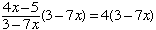Brandi Level 12 and above Student needing help 4x - 5 _____    =4 3-7x In word terms it would be 4x minus 5 divided by 3-7x = 4 and x cannot be three-sevenths Hi Brandi, I would first multiply both sides by 3 - 7x to getwhich simplifies to 4x - 5 = 12 - 28x Add 5 to both sides to get 4x = 17 - 28x and then add 28x to both sides 32x = 17 Finally divide both sides by 32 to get the result x = 17/32. Penny Go to Math Central